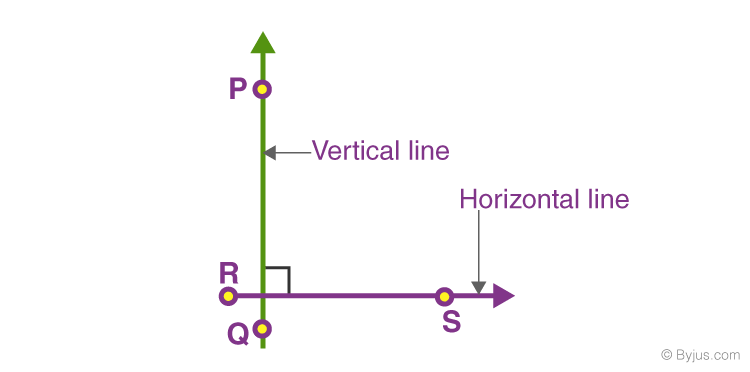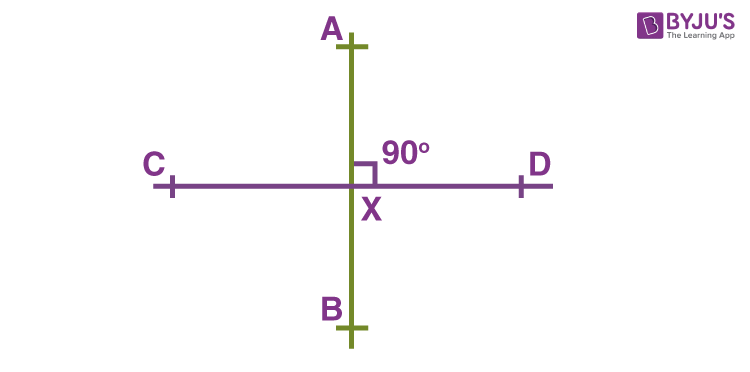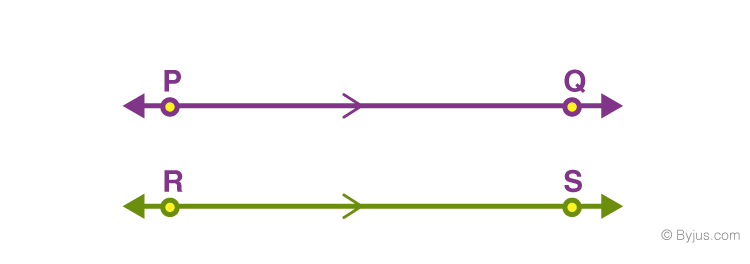# Perpendicular Lines

Perpendicular lines are formed when two lines meet each other at the right angle or 90 degrees. This property of lines is said to be perpendicularity. In this article, we are going to discuss what is perpendicular, the properties of perpendicular lines, the difference between perpendicular and parallel lines, and many solved examples in detail.

## What is Perpendicular?

In Mathematics, a perpendicular is defined as a straight line that makes the right angle (90 degrees) with the other line. In other words, if two lines intersect each other at the right angle, then the lines are perpendicular to each other. Now, consider the below figure. The line PQ and RS intersect each other at right angles and hence we can say that the lines PQ and RS are perpendicular to each other.## What is a Perpendicular Line?

The perpendicular lines are two lines that intersect each other and the angle formed between the two lines should be equal to 90 degrees (right angle). Consider the above-given figure, the line PQ and RS forms a right angle when the lines intersect at a point. Hence, the lines are perpendicular to each other and mathematically it is represented as PQ ⊥ RS.

When a line is perpendicular to the plane, then it is said to be perpendicular to all the points in the plane or perpendicular to each line in the plane that it intersects. Two planes are said to be perpendicular in space if the dihedral angle at which they meet the plane is a right angle.

Note: Perpendicular lines always intersect at 90 degrees but not all intersecting lines are perpendicular.

Also, read: Intersecting and Non-intersecting Lines

## Perpendicular Symbol

Perpendicular lines are represented by the symbol, ‘‘. Suppose, l1 and l2 are two lines intersecting each other at 90 degrees, then they are perpendicular to each other and are represented as l1⊥l2. The point of intersection is called the foot of the perpendicular.

## Properties of Perpendicular Lines

In mathematics, intersecting lines are not always perpendicular lines. The intersecting lines are said to be perpendicular lines if they obey the following properties. The two main properties of perpendicular lines are as follows:

• Perpendicular lines always intersect at the right angle
• If two lines are perpendicular to the same line, then they both are parallel to each other and never intersect.

## Slope of Perpendicular Lines

Suppose two lines AB and CD are perpendicular to each other. The slope of line AB is m1 and the slope of other line CD is m2.

Statement: Two lines are perpendicular to each other if and only if the product of the slope of the two lines equals minus of unity.

Thus, the formula for the slope of the perpendicular is given as:

 m1.m2 = -1

In the case of the parallel line, the slope of the two lines is parallel to each other.

m1 = m2

## Construction of Perpendicular Lines

Construction of the perpendicular line is a very simple process. The angle between the two lines should be equal to 90 degrees. So to construct perpendicular lines, you will need a compass and a straight line ruler or scale. Follow the below steps to draw it:

• Draw a horizontal line first.
• With the help of a compass draw an arc at the center of the line say point O, such that it intersects the line at two points and at equidistant from O. Let the two points be P and Q.
• Again at point P and Q, draw the arc inside, such that the two arcs intersect each other at the top and bottom of the horizontal line.
• Now join the two-point where the two arcs intersect each other.
• Now, the line obtained is perpendicular to the horizontal line.

## Examples

We know that if a ray is rotated about its end-point, the measure of its rotation is called the angle between its initial and final position. The value of any angle is proportional to its amount of rotation and the sense of its rotation. Clearly, the greater the amount of rotation, the larger will be the angle formed. A special case of angles is a right angle, in which the measure of rotation of a ray is 90o. When two lines or surfaces intersect to form right angles then such lines or surfaces are said to be perpendicular to each other.

Consider the following two-line segments

$$\begin{array}{l}\overline{AB}\end{array}$$
and
$$\begin{array}{l}\overline{CD}\end{array}$$
. These line segments are perpendicular to each other as they intersect at 90o at point X. Thus, both the line segments have a common intersection point i.e. X and are right angles to each other.Perpendicular lines lie in the same plane i.e. they are co-planar and intersect at right angles.  Thus it implies that if you have two lines that are perpendicular to each other, then these lines will be at right angles and vice versa.

Using just a compass one can draw a perpendicular to a line. These straightedge techniques were developed by ancient Greeks. In the case of co-ordinate geometry, a line is said to be perpendicular only if the slope of a line has a definite relationship.

### Real-Life Examples

If you simply look around you will find numerous examples of perpendicular lines and surfaces. The corners of the wall intersect each other at right angles, the tiles in the kitchen or the washroom, the intersection of roads at squares, hands of a clock when it strikes exactly three’ O clock,  the corners of your desk or the doors are examples illustrating perpendicularity.

### What are Parallel Lines?

In contradiction to perpendicular lines, in Geometry, we have parallel lines, which are parallel to each other and do not meet at any point.In the above image, the two lines PQ and RS are parallel to each other.

### Difference Between Parallel Lines and Perpendicular Lines

The difference between the parallel lines and perpendicular lines are given below:

 Parallel Lines Perpendicular Lines Parallel lines are the lines that do not intersect at any point and the distance between the two lines is always the same. Perpendicular lines are the lines that intersect each other at right angles (90 degrees). The symbol used to represent a parallel line is  “||”. The symbol used to represent perpendicular lines is  “⊥”. Example of Parallel lines: Opposite sides of a rectangle. Example of Perpendicular lines: Corner of two walls.

### Examples on perpendicular Lines

Example 1:

If one line passes through the points (0, –4) and (–1, –7) and another line passes through the points (3, 0) and (–3, 2). Are these lines parallel or perpendicular?

Solution:

Slope of first line,

m1 = (-7+4)/-1 = -3/-1 = 3

m2 = (2-0)/(-3-3) = 2/(-6) = -⅓

Since, m1 ≠ m2, therefore, lines are not parallel.

m1.m2 = 3 x (-⅓) = -1

Therefore, the two lines are perpendicular.

Example 2:

What is the equation for the line that is perpendicular to 4x−3y=6 through point (4,6)?

Solution:

As we know, the slope of perpendicular lines are opposite reciprocals.

Given, 4x−3y=6

Writing in slope-intercept form, we get;

y = (4/3)x – 2

So, slope of the line 4x−3y=6, m = 4/3

Now, the slope of line perpendicular to the given line is (-¾).

Using the coordinates of the point (4,6) and putting the given equation, we get;

6 = (-¾) (4) + b

b = 9

Therefore, the required equation of a perpendicular line is given by:

y = (-¾) x + 9

## Frequently Asked Questions on Perpendicular Lines

Q1

### What are perpendicular lines?

Perpendicular lines are those lines that intersect each other at 90 degrees.
Q2

### How are parallel lines different from perpendicular lines?

Parallel lines are parallel to each other and never intersect. But perpendicular lines intersect at right angle.
Q3

### Are all intersecting lines always perpendicular?

Not all intersecting lines are perpendicular to each other. They may intersect at different angles too.
Q4

### What is the condition for the slope of perpendicular lines?

If the slope of perpendicular lines are m1 and m2, then;
m1.m2 = -1
Q5

### Find the slope of a line perpendicular to the line y = –4x + 9.

Given, equation is y = -4x + 9
So, the slope of the given equation is -4.
Now the slope of the lines perpendicular to the given line is:
m = negative reciprocal of the slope of a given line
m = -1/(-4) = 1/4